## Monday, April 30, 2007

### Tileset Design Tip

Older games such as the early Ultimas had one tile for each type of terrain. Here's a screenshot of Ultima IV: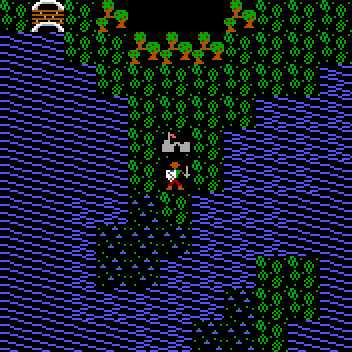The look of the terrain improves dramatically if we add transitions from one type of terrain to another. Here's Ultima V:Adding transitions can greatly increase the number of tiles required.

Here is a simple terrain consisting of two types of locations: ground and water. As in Ultima IV, there is one tile for each terrain type; the white lines indicate the boundaries of the tile bitmaps.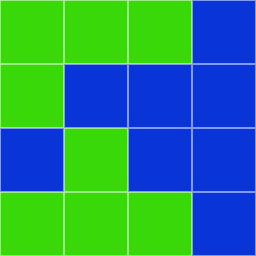To add artistic transitions, a natural progression from the Ultima IV-style tiles is to turn the ground tile into a set of ground tiles and the water tile into a set of water tiles. Each tile in a set corresponds to a different configuration of neighbor terrain types. This solution looks something like this: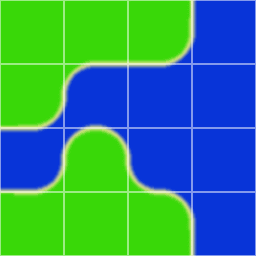The terrain types are effectively defined at the centers of the tiles. This is not a good solution; it creates a combinatorial explosion because the tile's appearance is affected by nine different grid locations.

It is much better to define terrain types at the corners of the tiles:Now each tile is defined by only four grid locations.

I got a comment about how foreground tiles should be aligned relative to the background tiles. The commenter is exactly right; foreground tiles are generally centered on the background tiles' corners. Here's an example with programmer art by me (except for the character which is from Zelda: Minish Cap):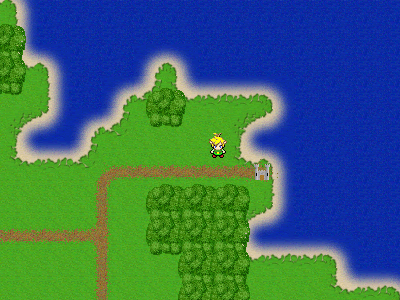The road, forest, and castle tiles are all offset so they are centered on the corners of the background tiles.

## Monday, April 23, 2007

### Hex grids

Geometry

A tiling of regular hexagons can be laid out on a rectangular grid:Via the Pythagorean Theorem, the relationship between horizontal and vertical grid line spacing is:For every hexagonal tiling there is an isomorphic triangular tiling representing hexagon adjacencies: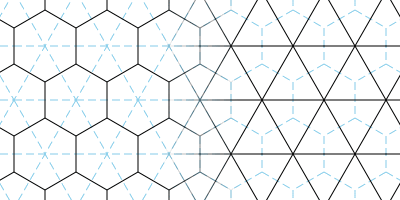Coordinates

How should coordinates be assigned to the hexagons in the grid? The first thing many people think of is to try to approximate the rows and columns of a rectangular grid:Transforming the isomorphic triangle grid to rectangular form shows the problem with this, though:Because the diagonals between adjacent hexes run in different directions on alternate rows, working with the hex grid is more complicated. For instance, a pathfinding routine needs to know the coordinates of each hex's neighbors. With this layout it is necessary to see if the hex is on an even row or an odd row, and generate a different set of neighbor coordinates for each.

A better way to number hexes is to keep both horizontal and “vertical” axes straight:Now, the transformation between the hexagonal layout and the square layout preserves all straight lines, and each hex looks like every other:It's fairly easy to convert back and forth between the two coordinate systems if the first one is desired from an interface standpoint.

Converting from plane coordinates to hex coordinates

How can a position in the continuous Cartesian plane be converted into hex grid coordinates? Examine this affine transformation:Four of each hex's edges now line up with the unit grid, and the two remaining diagonal edges are between hexes with the same X coordinate. Thus, every point within a square on the unit grid corresponds to the same X coordinate in the hex grid. After transforming the input point, round each component down (the floor() function in C) to get the integer coordinates of a unit grid square. From these unit grid square coordinates compute the hex X coordinate by summing the grid square X and Y, adding 2 (or whatever offset is necessary given your hex layout), and integer-dividing by 3, since the band of squares that correspond to the same hex X coordinate is 3 grid squares wide. In equation form:The hex Y coordinate is determined via a similar method. Using a different affine transform, once again align the hexes such that four of the hex edges are aligned with the unit grid, but this time ensure diagonal edges are between hexes with the same Y coordinate:Again, determine integer grid square coordinates by rounding down the transformed point's coordinates, then sum them, add 2, and integer-divide by 3 to yield the hex's Y coordinate.The precise A, B, C, and D coefficients for each of the transforms will depend on the scale and geometry of your hex grid.

To build a transformation matrix, think about what you want to come out when you put in (1, 0) and (0, 1). (1, 0) becomes (A, C) with our equations, while (0, 1) becomes (B, D).

Distance

Given the signed offset of a hex in x and y (in integer grid coordinates), it's easy to compute the number of steps needed to move to it. The formula is slightly different depending on which direction the grid's diagonals go.

If (1, 0) and (0, 1) are adjacent in the hex grid:If (0, 0) and (1, 1) are adjacent in the hex grid:References

Amit Patel has an excellent site covering lots of game-related topics, including a section on hex grids.

## Monday, April 16, 2007

### Aiming a projectile at a moving targetTo hit a moving target by shooting a gun you have to lead it, aiming at the spot where the target will be when the bullet reaches it. In this article we consider three successively harder versions of this problem.

To simplify the math, express the target's position and velocity relative to the shooter's, so that the shooter is effectively stationary at the origin. Assume that the target moves in a straight line, if it is moving. Also assume no air drag.

Motion without gravity

Without gravity, bullets travel in a straight line at constant velocity. To determine where to shoot, imagine shooting an infinite number of bullets simultaneously in all directions, forming an expanding sphere of death. While the sphere is expanding, the target is moving along a straight line (or standing still). If we can determine the instant at which this expanding sphere touches the moving target, we know where to aim: at the target's position when it gets hit by the expanding sphere of death.

Variable definitions:Equation for the time when the target and bullet are equidistant from the shooter:Square both sides:Expand: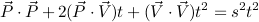Group terms by powers of t:Examine the equation above. If the target's velocity is zero, the impact time works out to simply be target distance divided by bullet speed, which makes sense. The constant term is never negative; it is positive as long as shooter and target are not at the same position. The quadratic term curves upward if the target's speed is faster than the bullet speed. In this case, only the linear term would be capable of bringing the curve below the zero line. In problem terms: if the target is faster than the bullet, the bullet can hit the target only if the target is moving toward the shooter fast enough.

Code for solving this quadratic might look like this:
``double time_of_impact(double px, double py, double vx, double vy, double s){    double a = s * s - (vx * vx + vy * vy);    double b = px * vx + py * vy;    double c = px * px + py * py;    double d = b*b + a*c;    double t = 0;    if (d >= 0)    {        t = (b + sqrt(d)) / a;        if (t < 0)            t = 0;    }    return t;}``
I've done a bit more simplification in going from the equation to the code. Any time there's a 2 on the linear coefficient of the quadratic (which actually happens fairly frequently in my experience), it cancels with the 2 in the denominator and the 4 in the discriminant of the usual quadratic formula. Also, negating the quadratic coefficient (a in the code) cleans out a few minus signs.

Once t is known, plug it into the expression for the motion of the target to find the point to aim at: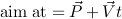This code works equally well with 3D vectors. In a real game I'd be using a vector data type rather than passing around the X, Y, and Z components individually, and I'd have a function for doing dot products instead of writing out x1*x2 + y1*y2 + z1*z2 every time.

If the discriminant (d in the code) is less than zero, it means there are no real roots. In real terms this means the target is moving away faster than the bullet can travel, so there is no possibility of an impact. The code deals with the no-root situation by clamping t to zero, which will cause the gun to aim at the target's current position. A more sensible thing to do might be to find the time t that minimizes distance between bullet and target, and aim at the target's position then.

There is also generally a negative root which corresponds to playing time backwards from the current moment, and as such is not usable.

Space combat games such as Freelancer or Darkstar One draw a targeting reticle ahead of enemy ships, at the spot where you need to shoot in order to hit them. Now you can go forth and do likewise. Obviously, the aim position is different for different bullet speeds, so if you have turbolasers that shoot at 4.5 km/sec and linear tachyon atomizers that shoot at 6 km/sec, you might want two reticles.

Gravity without motion

On earth, gravity pulls bullets downward as they fly. (They also slow down quite a bit due to air resistance, but I'm not going to get into that today.) To counteract gravity you have to aim higher for far-away targets. Let's figure out how to do this with a stationary target first.

Returning to our expanding sphere of death, we could imagine it falling due to gravity as it expands. Equivalently, we could think of the stationary target “falling” upward into the sky instead. This allows us to leave the sphere centered on the origin. Here's the equation for a target which starts at rest and falls upward until it hits the expanding sphere:In a typical game, gravity operates along a single axis. In this case, the G vector will have zeros in all the other components. Squaring: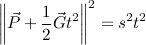Expand:Group terms by power of t:Although we have a fourth power of t, we can substitute u = t2 and be left with a quadratic instead:The two solutions to this u quadratic correspond to the two parabolic arcs that pass through the target point. There's a short, low arc and a longer, high arc. (At maximum range they merge together into one.) Choose whichever one works best for your situation.

Once you've solved for t, plug it into the equation for motion of the target to get the aim position: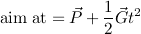Be sure that your targeting code integrates to the same position your simulation will when it moves the bullet through the air. The equations above assume correct integration, but as a kid I wrote things that weren't quite right. For instance:
`newPosition = oldPosition + oldVelocity;newVelocity = oldVelocity + acceleration;`
The code is assuming that velocity is expressed in distance per frame, and acceleration in distance per frame squared. However, it should be adding half the acceleration to the position as well, like this:
`newPosition = oldPosition + oldVelocity + 0.5 * acceleration;newVelocity = oldVelocity + acceleration;`

Gravity and motion together

Finally, let's shoot an arcing projectile at a moving target! The initial equation is just a combination of the starting equations for the previous two cases:Square:Expand: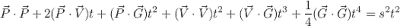Collect terms by power of t:This is a quartic equation and there's no way around it. Let's start by sanity-checking it.

If gravity is zero, the quartic and cubic terms drop out (as well as part of the quadratic term) and we're left with the motion-but-no-gravity equation. If velocity is zero instead, the cubic and linear terms drop out (as well as part of the quadratic term) and we're left with the gravity-but-no-motion equation.

When I'm working on solving problems like this, I find it helpful to rig up my game to plot graphs in real time. In this case, I drive the target around and get a constantly-updated graph of the polynomial and its derivatives for shooting at it. This allows me to quickly get a feel for the nature of the problem. Here's an example graph (with added labels):Looking at the equation, you can see that the fourth-order term will always be positive, which means that the red curve will always turn upward on the outer ends. The constant term will always be positive as long as the target is not at the same place as the shooter, which means the curve will be positive as it crosses the Y axis.

The quartic curve has (up to) three points where it changes direction from upward to downward or vice versa. One way to find the roots would be to divide the curve up into intervals between these points. If one end of an interval is above zero and the other is below zero, you know there is a root within that interval. You could use a binary search to locate it, or you could use the midpoint of the interval as an initial guess and use Newton's method to converge on the root. The outer intervals are unbounded so binary search wouldn't necessarily work there, but you could double a t value until it evaluates positive and then binary search within that interval.

The extrema of the quartic correspond to zeros (roots) of its first derivative (the green line in the graph). The first derivative of a quartic is a cubic. We could follow a similar procedure to find its roots: find the zeros of its derivative (the blue line); use them to subdivide the curve into intervals; evaluate the function at the interval endpoints; and search for roots in the intervals that cross the X axis.

When there are no roots, I decided I wanted my gun to shoot at an angle that brought the bullets as close as possible to the target. This corresponds to finding the non-negative t that gives the smallest value for the quartic function. Minima have to be either at one of the zeros of the first derivative, or at t = 0. Once the roots of the cubic are known it's simple to evaluate the function at them, and at t = 0, and pick the one with the smallest value.

Jochen Schwarze wrote some cubic/quartic solving code in Graphics Gems I which works great.

Once again, once t is known, plug it into the left-hand side of the original equation to get the aim position: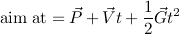Ron Levine wrote a Usenet posting a few years ago covering some of the same ground. There's also a page on the Unreal Wiki.

## Monday, April 9, 2007

### Raytracing on a grid, in Haskell

I attempt to write something in Haskell every now and then. Mainly this is because I think we're going to have to move beyond the imperative model in order to effectively use our increasingly parallel computers. Languages like C are built around the assumption that there is a single locus of control which is reading data from memory, modifying it, and writing it back. Multi-threaded programming is possible in C but it's devilishly difficult.

Haskell's a good-looking language, in my opinion; it is parenthesized like Scheme, but due to its equational and algebraic syntax needs far less of them. It uses layout like Python to indicate block structure, which eliminates even more syntactic verbiage. It's got static typing with type inference, so the compiler catches my stupid mistakes with a minimum of additional typing. In general, when the program compiles, it works, which is a very different experience from coding in, say, Scheme or Python.

For fun, I ported my grid raytracing test program from C++ to Haskell. Here's the original C++ code:

``#include <cmath>#include <windows.h>#include <gl/glut.h>static void draw_frame();static void window_was_resized(int width, int height);static void key_pressed(unsigned char key, int x, int y);static void mouse_activity(int button, int state, int x, int y);static const initial_window_size_x = 800;static const initial_window_size_y = 800;static const pixels_per_unit = 64;static int g_window_width  = 0;static int g_window_height = 0;static double g_line_x0 = 4;static double g_line_y0 = 1;static double g_line_x1 = 1;static double g_line_y1 = 1;static int g_nr_points_defined = 2;int main(int argc, char * argv[]){    glutInit(&argc, argv);    glutInitDisplayMode(GLUT_RGB | GLUT_DOUBLE);    glutInitWindowPosition    (        (GetSystemMetrics (SM_CXSCREEN) - initial_window_size_x) / 2,        (GetSystemMetrics (SM_CYSCREEN) - initial_window_size_y) / 2    );    glutInitWindowSize(initial_window_size_x, initial_window_size_y);    glutCreateWindow("Line Draw Test");    glutReshapeFunc(window_was_resized);    glutDisplayFunc(draw_frame);    glutKeyboardFunc(key_pressed);    glutMouseFunc(mouse_activity);    glEnable(GL_COLOR_MATERIAL);    glutMainLoop();    return 0;}static void plot_squares(double x0, double y0, double x1, double y1){    double dx = fabs(x1 - x0);    double dy = fabs(y1 - y0);    int x = int(floor(x0));    int y = int(floor(y0));    int n = 1;    int x_inc, y_inc;    double error;    if (x1 > x0)    {        x_inc = 1;        n += int(floor(x1)) - x;        error = (ceil(x0) - x0) * dy;    }    else    {        x_inc = -1;        n += x - int(floor(x1));        error = (x0 - floor(x0)) * dy;    }    if (y1 > y0)    {        y_inc = 1;        n += int(floor(y1)) - y;        error -= (ceil(y0) - y0) * dx;    }    else    {        y_inc = -1;        n += y - int(floor(y1));        error -= (y0 - floor(y0)) * dx;    }    glColor3d(0.25, 0.25, 0.5);    glBegin(GL_QUADS);    for (; n > 0; --n)    {        glVertex2i(x,   y  );        glVertex2i(x+1, y  );        glVertex2i(x+1, y+1);        glVertex2i(x,   y+1);        if (error > 0)        {            y += y_inc;            error -= dx;        }        else        {            x += x_inc;            error += dy;        }    }    glEnd();}static void draw_frame(){    glClear(GL_COLOR_BUFFER_BIT);    // Set up the projection matrix.    double window_world_size_x = double(g_window_width ) / pixels_per_unit;    double window_world_size_y = double(g_window_height) / pixels_per_unit;    glMatrixMode(GL_PROJECTION);    glLoadIdentity();    gluOrtho2D(0, window_world_size_x, 0, window_world_size_y);    // Plot the line's squares if the line is defined.    if (g_nr_points_defined >= 2)    {        plot_squares(g_line_x0, g_line_y0, g_line_x1, g_line_y1);    }    // Draw a background grid.    int number_of_lines_x = int(ceil(window_world_size_x));    int number_of_lines_y = int(ceil(window_world_size_y));    glColor3d(0.5, 0.5, 0.5);    glBegin(GL_LINES);    int i;    for (i = 0; i < number_of_lines_x; ++ i)    {        glVertex2d(i, 0);        glVertex2d(i, window_world_size_y);    }    for (i = 0; i < number_of_lines_y; ++ i)    {        glVertex2d(0, i);        glVertex2d(window_world_size_x, i);    }    glEnd();    // Draw the actual line, if defined.    if (g_nr_points_defined == 1)    {        glColor3d(1, 1, 1);        glBegin(GL_POINTS);        glVertex2d(g_line_x0, g_line_y0);        glEnd();    }    else if (g_nr_points_defined >= 2)    {        glColor3d(1, 1, 1);        glBegin(GL_LINES);        glVertex2d(g_line_x0, g_line_y0);        glVertex2d(g_line_x1, g_line_y1);        glEnd();    }    glutSwapBuffers();}static void window_was_resized(int width, int height){    g_window_width  = width;    g_window_height = height;    glViewport(0, 0, width, height);}static void key_pressed(unsigned char key, int x, int y){    if (key == 27)    {        exit (0);    }}static void mouse_activity(int button, int state, int x, int y){    if (state == GLUT_DOWN)    {        if (button == GLUT_LEFT_BUTTON)        {            // Get the coordinates of the mouse click in world coordinates.            GLdouble modelview_matrix;            GLdouble projection_matrix;            GLint viewport;            glGetDoublev(GL_MODELVIEW_MATRIX, modelview_matrix);            glGetDoublev(GL_PROJECTION_MATRIX, projection_matrix);            glGetIntegerv(GL_VIEWPORT, viewport);            double wx, wy, wz;            gluUnProject(x, (g_window_height - 1) - y, 0, modelview_matrix,                projection_matrix, viewport, &wx, &wy, &wz);            if (g_nr_points_defined == 1)            {                g_line_x1 = wx;                g_line_y1 = wy;                g_nr_points_defined = 2;            }            else            {                g_line_x0 = wx;                g_line_y0 = wy;                g_nr_points_defined = 1;            }            glutPostRedisplay();        }    }}``

Here is the same program written in Haskell:

``-- Raytracing on a gridimport Data.IORef (IORef, newIORef, readIORef, modifyIORef)import System.Exit (exitWith, ExitCode(ExitSuccess))import Graphics.Rendering.OpenGLimport Graphics.UI.GLUTinitialWindowSizeX = 800initialWindowSizeY = 800pixelsPerUnit = 64main = do    getArgsAndInitialize    initialDisplayMode \$= [RGBAMode, DoubleBuffered]    initialWindowSize \$= Size initialWindowSizeX initialWindowSizeY    (Size screenSizeX screenSizeY) <- get screenSize    let initialPos = Position x y where        x = (screenSizeX - initialWindowSizeX) `div` 2        y = (screenSizeY - initialWindowSizeY) `div` 2    initialWindowPosition \$= initialPos    createWindow "Grid Raytracing Demo"    endpoints <- newIORef []    displayCallback \$= display endpoints    reshapeCallback \$= Just reshape    keyboardMouseCallback \$= Just (keyboard endpoints)    matrixMode \$= Projection        mainLoopdisplay endpoints = do    clear [ColorBuffer]    points <- readIORef endpoints    windowPixelSize <- get windowSize    drawSquares points    drawGrid windowPixelSize    drawLine points    swapBuffersreshape size@(Size w h) = do    viewport \$= (Position 0 0, size)    loadIdentity    ortho2D 0 ((fromIntegral w) / pixelsPerUnit) 0 ((fromIntegral h) / pixelsPerUnit)keyboard _ (Char '\27') Down _ _ = exitWith ExitSuccesskeyboard endpoints (MouseButton LeftButton) Down _ pos = do    (wx, wy) <- worldFromScreen pos    modifyIORef endpoints (addEndpoint (wx, wy))    postRedisplay Nothingkeyboard _ _ _ _ _ = return ()addEndpoint pos (_:_:_) = [pos]addEndpoint pos endPoints = pos : endPointsdrawSquares (end1 : end0 : _) = do    currentColor \$= Color4 0.25 0.25 0.5 1    renderPrimitive Quads \$ mapM_ drawQuad (squaresOnLine end0 end1)    where        drawQuad (x, y) = do            vertex \$ Vertex2 x0 y0            vertex \$ Vertex2 x1 y0            vertex \$ Vertex2 x1 y1            vertex \$ Vertex2 x0 y1            where                x0 :: GLint                x0 = fromIntegral x                x1 = fromIntegral x + 1                y0 = fromIntegral y                y1 = fromIntegral y + 1drawSquares _ = return ()drawGrid (Size sizeX sizeY) = do    currentColor \$= Color4 0.5 0.5 0.5 1    renderPrimitive Lines \$ mapM_ (\(x, y) -> vertex \$ Vertex2 x y) points    where        points = (interleave minYs maxYs) ++ (interleave minXs maxXs)        minXs = zip (repeat 0) ys        maxXs = zip (repeat maxX) ys        minYs = zip xs (repeat 0)        maxYs = zip xs (repeat maxY)        xs = take (ceiling maxX) [0..]        ys = take (ceiling maxY) [0..]        maxX = (fromIntegral sizeX) / pixelsPerUnit        maxY = (fromIntegral sizeY) / pixelsPerUnitinterleave (x:xs) (y:ys) = x : y : interleave xs ysinterleave _ _ = []drawLine ((x0, y0) : (x1, y1) : _) = do    currentColor \$= Color4 1 1 1 1    renderPrimitive Lines \$ do        vertex \$ Vertex2 x0 y0        vertex \$ Vertex2 x1 y1drawLine ((x0, y0) : _) = do    currentColor \$= Color4 1 1 1 1    renderPrimitive Points \$ do        vertex \$ Vertex2 x0 y0drawLine _ = return ()worldFromScreen (Position sx sy) = do    viewport@(_, Size _ viewSizeY) <- get viewport    projectionMatrix <- get (matrix \$ Just Projection) :: IO (GLmatrix GLdouble)    modelviewMatrix <- get (matrix \$ Just \$ Modelview 0) :: IO (GLmatrix GLdouble)    let screenPos = Vertex3 (fromIntegral sx) (fromIntegral ((viewSizeY - 1) - sy)) 0    (Vertex3 wx wy wz) <- unProject screenPos projectionMatrix modelviewMatrix viewport    return (wx, wy)squaresOnLine (x0, y0) (x1, y1) = take n (genSquares (floor x0) (floor y0) error) where    n = 1 + abs((floor x1) - (floor x0)) + abs((floor y1) - (floor y0))    dx = abs (x1 - x0)    dy = abs (y1 - y0)    xInc = if x1 > x0 then 1 else -1    yInc = if y1 > y0 then 1 else -1    error = dy * tNextX - dx * tNextY    tNextX        | x1 > x0   = (fromIntegral (ceiling x0)) - x0        | otherwise = x0 - (fromIntegral (floor x0))    tNextY        | y1 > y0   = (fromIntegral (ceiling y0)) - y0        | otherwise = y0 - (fromIntegral (floor y0))    genSquares x y error        | error > 0 = (x, y) : genSquares x (y + yInc) (error - dx)        | otherwise = (x, y) : genSquares (x + xInc) y (error + dy)``

Both versions use OpenGL with the GLUT library for handling window creation/resizing and keyboard/mouse input. The Haskell version clocks in at around 126 lines, while the C++ version has around 230 lines. I have much more experience writing C++ than Haskell, so the Haskell version may not be great style.

Obvious differences:

Haskell, like Python, uses indentation to indicate the block structure of the code, instead of explicit markers. In C++ I prefer to keep my block markers lined up vertically to aid in matching them visually; this adds lines to the code.

The C++ code contains much more type annotation than the Haskell code. (Haskell type annotations are the phrases beginning with :: such as :: GLint.) Because types are largely inferred, this Haskell program contains only three type annotations. These are needed because Haskell's OpenGL binding is parameterized on input types, so for instance the Vertex2 constructor can take integer, 32-, or 64-bit floating point values as input. Because the input numbers don't offer any clues, the compiler needs a hint to know which one to use. If the constructors for the different types were named differently (Vertex2i, Vertex2f, and Vertex2d, say, as is done in the C API) then these type annotations would not be necessary.

C++ inherits C's single-pass compilation model to some extent, so forward declarations are necessary to be able to call functions before the lines where they are defined. Haskell allows you to define and use functions in any order.

Because OpenGL is implemented as a state machine, and because of the nature of input and output in general, most of the Haskell code has an imperative structure. In fact, the only functions that are purely functional (meaning all inputs and outputs are explicitly listed) are addEndpoint, interleave, and squaresOnLine.

Conditionals tend to be represented without if-statements in the Haskell code. Some if statements transform into multiple function definitions, with each definition covering a portion of the possible input. For instance, drawLine draws nothing if no endpoints are defined; draws a single point if only one endpoint has been defined; and draws a line between endpoints otherwise. Other if statements transform into patterns on a single function definition. For instance, tNextX in squaresOnLine is defined differently depending on the relative magnitudes of x0 and x1.

Loops, likewise, are less obvious in the Haskell version. The first line of squaresOnLine calls take to take the first n items of a list, for instance. It gets an infinite list from the genSquares subfunction which uses recursion to produce the list. Recursion is the heart of how loops are handled in Haskell, but often it's possible to call a function to handle the recursion internally. The mapM_ in drawGrid is an example of this.

Some less obvious differences:

The Haskell program separates concerns better. For instance, the squaresOnLine function generates all the squares intersected by the line segment, but drawing those squares is done in drawSquares. If we need to find the squares to do something else it is easy to reuse squaresOnLine. This could (and probably should) be done in the C++ version, but would likely add a fair amount of additional code.

Internal functions are extremely handy. Note that genSquares inside squaresOnLine can see the variables dx, dy, xInc, and yInc defined in the outer function. This simplifies the interface to genSquares so that it only contains the values that change during generation.

Haskell has no implicit conversion from integer to floating-point types, so lots of fromIntegral calls are needed.

Managing mutable state is kind of a pain. Fortunately for this program, the only state we have is the endpoints that the user has chosen. The program creates a mutable variable (an “IORef”) at startup, modifies it when the user clicks the mouse, and reads it to draw the view.

## Monday, April 2, 2007

### Notes on Outcast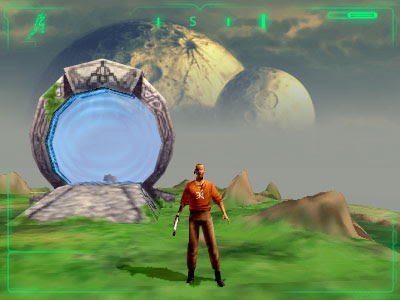Outcast was a remarkable game produced by the now-defunct Belgian studio Appeal and published by Infogrames (now Atari) in 1999. If you've seen the movie Stargate you have the gist of the game's plot. Your character is transported to a network of worlds connected by stargates (sorry, daokas). These worlds are populated by humanoid aliens who have learned some English (if you've seen the movie you have an idea why), and are ruled by an oppressive tyrant. It seems everyone has been expecting your arrival for many years, and you are thrust into the role of a messiah. It's a lengthy action-adventure, so you can expect much combat, conversation, and carrying of items.

Outcast was released during the early days of hardware 3D acceleration, when accelerators were capable of pushing lots of pixels but not much else. 3D-accelerated games tended to all look pretty similar. Rather than go down the hardware-acceleration road, Appeal opted to use a software renderer. This allowed them to produce gorgeous effects that would not be possible in hardware rendering for several more years. These effects included things like depth-of-field blurring, bump mapping, and environment-mapped water reflections. The terrain was rendered using raycasting (a restricted form of raytracing); doing a terrain with the same density of detail using polygons is a bit of a challenge even today.

Unfortunately, the graphic effects came at a heavy cost: you needed a top-of-the-line PC at the time, but it rendered to sub-640x480 resolutions. These ended up limiting the market of people who wanted to play the game, as gamers with high-end PCs also tended to have 3D accelerators that they wanted to see put to use.

The soundtrack is sumptuous, featuring both the Moscow Symphony and Choir. The songs are wonderful. Dialogue is spoken throughout; the games uses cell-phone GCM compression and so is able to fit vast amounts of speech into a modest amount of disk space.

Outcast made some interesting design decisions that I want to mention:

The aliens had their own language before being introduced to English. Like most situations where a conqueror's language is superimposed on a native language, lots of nouns remain from the native language, and the aliens will occasionally talk to each other entirely in their own language, leaving you feeling somewhat left out. In Outcast you are overwhelmed at first by the new vocabulary, but the game provides you with a lexicon that fills in as you are introduced to new words, and soon you know all about daokas and the rest. I found this to be quite immersive; the designers went the extra mile to make the world feel plausible.

Innumerable games have numbers representing the player's strength, dexterity, and so forth, which improve over the course of the game. Rather than the player character getting stronger or faster, Outcast gives you missions to complete which weaken the enemies. For instance, you can cut off their food supplies, sabotage their weapons production, or cut off their pay. These have different, specific effects: cutting off food reduces their hit points, sabotaging weapons reduces the amount of damage they do, and cutting pay reduces the overall number of soldiers you can encounter. I like this approach very much.

Being an adventure game, there are many, many times when you need to travel somewhere to talk to someone. The game world is fairly densely populated. Some of these characters have names and are important to the game plot; the majority of them are “extras” and can only provide general information. Finding a specific named person among the crowds could be a major challenge, but the designers provided a fantastic mechanism that I am still amazed few other games have done: you can stop and ask for directions.

When you strike up a conversation with someone, one of the things you can ask is “Where's So-and-so?” Depending on how far away the target is, you will get different answers. If the target is in a different world, the person will tell you which one. Once you're in the right world but far away, you will get an indication of the general area the target occupies. As you get closer you get more precise answers, until you are within sight; then the person you are talking to will simply turn and point, saying “That's him, over there.”

The behavior of people in general is quite good. They have business to do: moving boxes, harvesting riss, delivering water, begging, mining, or collecting taxes, to name just a few activities. When gunfights erupt they cower or run. Soldiers take cover and step out to fire. Their leaders will call them to arms, and they circle around to try and get crossfire on you.

To conclude: I'd highly recommend trying out this game if you can get your hands on it. It runs fine on modern computers with a couple of exceptions. Movement through rice paddies is excruciatingly slow (instead of being merely slow, as intended), and mounted riding on twonhas is problematic; they tend to get stuck. I don't remember either of these problems when playing it originally, so it probably has something to do with the vastly higher clock speeds modern computers have. Neither of these problems is a game-breaker.

Addendum: I wrote the post from memory, not having played Outcast in a couple of years. I fired it up tonight to grab a screenshot. Unfortunately it doesn't run so well on my current computer; it crashes consistently when I attempt to travel through the portal shown above. Hopefully your luck will be better. Outcast does some interesting things with its data files (such as the GSM compression for voice) which I hope to cover in a future article.

Next Monday: Grid raytracing code in Haskell